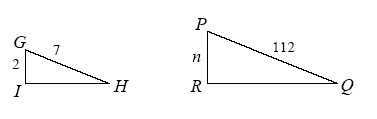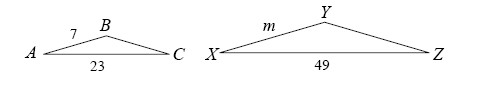### Home > GC > Chapter 3 > Lesson 3.2.3 > Problem3-71

3-71.

Solve for the missing lengths in the sets of similar figures below. Show all work.

See the Math Notes box in Lesson 3.1.3 for extra help.

 $ΔGHI ∼ ΔPQR$Step 1(a):$\frac{2}{7} = \frac{n}{112}$
 $ΔABC ∼ ΔXYZ$Hint (b):Use the same method you used to solve part (a).